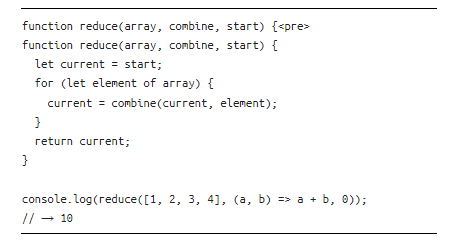# <pre> instruction in function

This is a code snippet from Eloquent JavaScript in its Chapter 5 about Higher Order Functions:

``````
function reduce(array, combine, start) {<pre>
function reduce(array, combine, start) {
let current = start;
for (let element of array) {
current = combine(current, element);
}
return current;
}

console.log(reduce([1, 2, 3, 4], (a, b) => a + b, 0));

// → 10
``````

I can understand the logic of this snippet but I have one question: What’s with the `<pre>` ? What does it do?

In the example he uses the array `[1, 2, 3, 4]` and the `combine` function he defines an arrow function that adds elements. At the beginning `current`is equal to the `start` value defined in function call. Then the code defines `current` as the `combine` function of `current` and `element` from the array looping through it to finally return `current`. Swell…

Yet, I don’t understand what’s with the instruction `<pre>`… Is this some sort of recursion?

This does not make any sense. You would only have a single function definition (the line below what shows above). I looked at chapter 5 but do not see the code you show above anywhere. Can you add a link to the specific code or take a screenshot of it?

I see the following code under the Reduce section.

1 Like

You must (somehow) have copied some HTML out. It isn’t part of the code.

If you click on the code it will open an editor view and you can actually run the code using the little menu on the top right. I would suggest you copy code from that view.

1 Like

Many thanks. Indeed, it didn’t make any sense and the one that you show from the website makes sense. This is from the ebook that I have:The code runs OK in the book’s website…